Function Repository Resource:

# IntegerPartitionQ

Check whether the argument is a weakly decreasing list of positive integers

Contributed by: Wolfram Staff
 ResourceFunction["IntegerPartitionQ"][x] checks whether x is a weakly decreasing list of positive integers. ResourceFunction["IntegerPartitionQ"][x,n] checks whether x is an integer partition of n.

## Details

An integer partition is a multiset of positive integers and so not ordered. Therefore, any order can be used to represent it. Typically, the order chosen is weakly decreasing, as here; some people choose weakly increasing.

## Examples

### Basic Examples (2)

Check an integer partition:

 In:=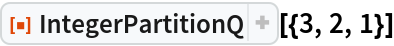Out=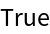Check whether it is an integer partition of 6:

 In:=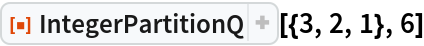Out=Here are the 5 integer partitions of 4:

 In:=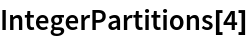Out=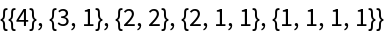They all pass the test for being an integer partition:

 In:=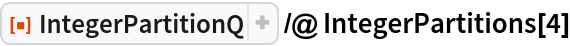Out=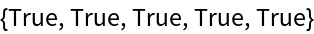### Properties and Relations (3)

The parts all have to be integers:

 In:=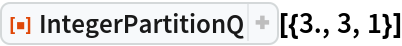Out=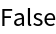In:=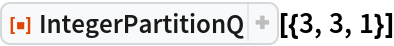Out=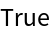The parts have to be positive:

 In:=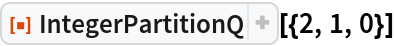Out=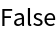In:=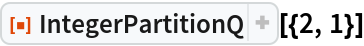Out=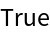The parts have to be in weakly decreasing order:

 In:=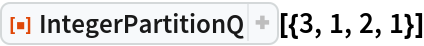Out=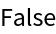In:=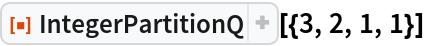Out=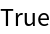George Beck

## Requirements

Wolfram Language 11.3 (March 2018) or above

## Version History

• 1.0.1 – 31 January 2022
• 1.0.0 – 19 April 2019